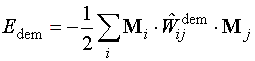### Theoretical background

I. Basic micromagnetic concept and main energy contributions

Theoretical micromagnetics as founded by W.F.Brown is a phenomenology for the evaluation of the total magnetic free energy Etot of a ferromagnetic body if its geometry, material parameters and the magnetization configuration are known. In its 'minimal' version micromagnetics takes into account four energy contributions: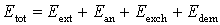Energy in the external field Eext: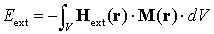Magnetocrystalline anisotropy energy Ean (e.g., uniaxial case):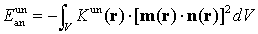Exchange stiffness energy Eexch (isotropic exchange assumed):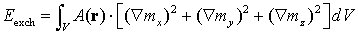Magnetodipolar interaction energy (stray or demagnetizing) field energy Edem: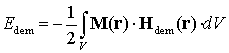where the demagnetizing field Hdem can be calculated as a convolution of the magnetization distribution inside a ferromagnet with the dipolar interaction kernel.

II. Finite-difference approximations of the energy contributions used in MicroMagus

Notation:

Mi - magnetization in the cell # i, mi = Mi/Mi
Hiext - external field averaged over the cell # i
DVi - cell volume

1. External field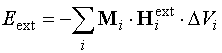2. Anisotropy (Ki ñ anisotropy constants)
• uniaxial (ni ñ unit vector of the anis. axis)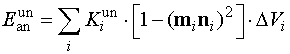• cubic (px,y,z ñ components of the unit magn. vector in a local coord. system)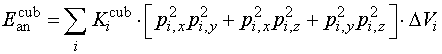3. Exchange (Jij ñ exchange coeff., kij ñ exch. weakening, aij ñ angle between Mi and Mj)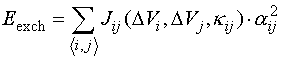4. Stray field (Wij ñ interaction coeff. between cells i and j)